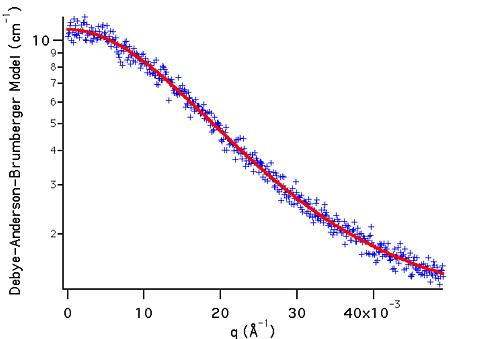MODEL

Debye-Anderson-Brumberger (DAB)

AUTHOR/MODIFICATION

Alan Munter 08 JULY 1999, converted to Java

APPROVED FOR DISTRIBUTION

DESCRIPTION

Calculates the scattering from a randomly distributed (i.e. nonparticulate), two-phase system based on the Debye-Anderson-Brumberger (DAB) model for such systems. The two-phase system is characterized by a single length scale, the correlation length, which is a measure of the average spacing between regions of phase 1 and phase 2. The model also assumes smooth interfaces between the phases and hence exhibits Porod behavior (I ~ Q-4) at large Q (Q*correlation length >> 1). The macroscopic scattering cross-section in the DBA model is given by

dS/dW = A/(1 + (q*a)^2)^2

where a is the correlation length in Å.

Resolution smeared version is also provided.

VARIABLES

Input Variables (default values):

Parameter Variable Value
0Scale10.0
2Incoherent Background (cm-1)0.0

USAGE NOTES

The returned value is in units of [cm-1].

The scale factor, A or Parameter, is treated as an independent fitting parameter, but is, in the DBA model, related to the volume fractions of the two phases, f1 and f2, their contrast, (sld_1 - sld_2)2, and the correlation length, a, as follows:

A = 8*p*a3*contrast*f1*f2

The surface, S, to volume, V, ratio for the system is given by

S/V = 4*f1*f2/ a

REFERENCES

Debye, P., Anderson, R., Brumberger, H., "Scattering by an Inhomogeneous Solid. II. The Correlation Function and Its Application," J. Appl. Phys. 28 (6), 679 (1957).

Debye, P., Bueche, A. M., "Scattering by an Inhomogeneous Solid," J. Appl. Phys. 20, 518 (1949).

TEST DATASET

This example dataset is produced by multiplying the values generated by the Model DAB (using the default parameter values given above) by (1 + gnoise(.05)) to simulate real data with 5% gaussian noise. The q-range has been restricted to (0.001,0.05) Å-1.